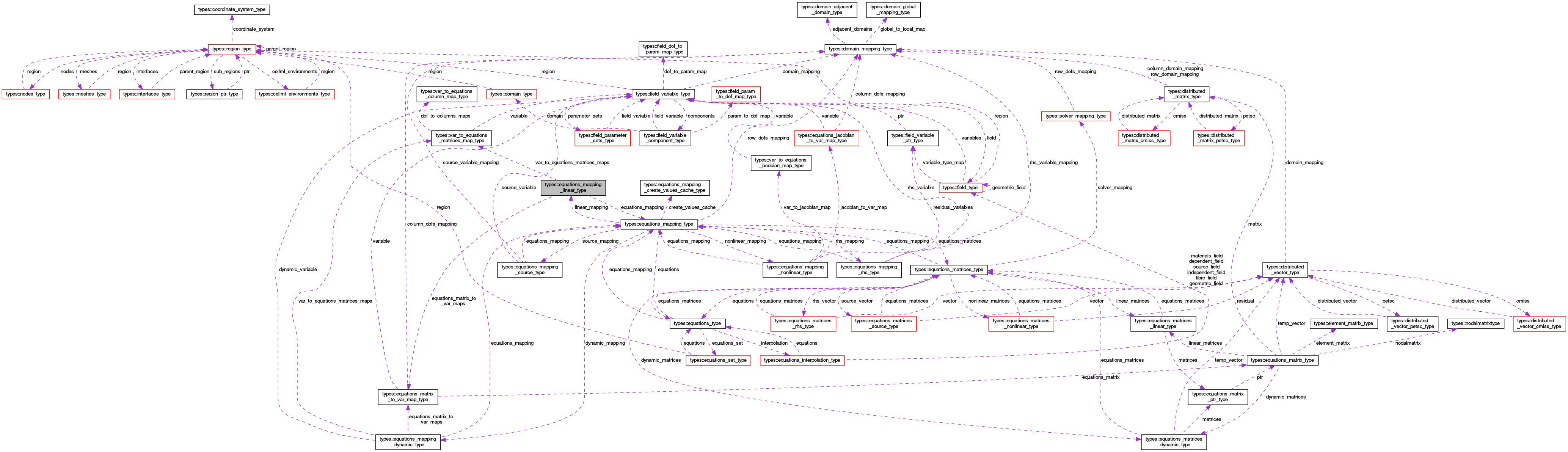OpenCMISS-Iron Internal API Documentation
types::equations_mapping_linear_type Type Reference

Contains information for mapping field variables to the linear matrices in the equations set of the mapping. More...

Collaboration diagram for types::equations_mapping_linear_type:[legend]

## Public Attributes

type(equations_mapping_type), pointer equations_mapping
A pointer to the equations mapping. More...

integer(intg) number_of_linear_equations_matrices
The number of linear equations matrices in this mapping. More...

integer(intg) number_of_linear_matrix_variables
The number of dependent variables involved in the linear equations matrix mapping. More...

integer(intg), dimension(:), allocatable linear_matrix_variable_types
LINEAR_MATRIX_VARIABLE_TYPES(i). The variable type of the i'th variable type involved in the equations linear matrix mapping. TODO: just make this the size of the number of matrix variables rather than the field number of variable types and merge matrix variable types above??? More...

type(var_to_equations_matrices_map_type), dimension(:), allocatable var_to_equations_matrices_maps
VAR_TO_EQUATIONS_MATRICES_MAPS(variable_type_idx). The equations matrices mapping for the variable_type_idx'th variable type. More...

type(equations_matrix_to_var_map_type), dimension(:), allocatable equations_matrix_to_var_maps
EQUATIONS_MATRIX_TO_VAR_MAPS(matrix_idx). The mappings for the matrix_idx'th equations matrix. More...

integer(intg), dimension(:,:), allocatable equations_row_to_variable_dof_maps
EQUATIONS_ROW_TO_VARIABLE_DOFS_MAPS(row_idx,variable_type_idx). The row mappings for the row_idx'th row of the equations matrices to the variable_type_idx'th variable. More...

## Detailed Description

Contains information for mapping field variables to the linear matrices in the equations set of the mapping.

Definition at line 1587 of file types.f90.

## Member Data Documentation

 type(equations_mapping_type), pointer types::equations_mapping_linear_type::equations_mapping

A pointer to the equations mapping.

Definition at line 1588 of file types.f90.

 type(equations_matrix_to_var_map_type), dimension(:), allocatable types::equations_mapping_linear_type::equations_matrix_to_var_maps

EQUATIONS_MATRIX_TO_VAR_MAPS(matrix_idx). The mappings for the matrix_idx'th equations matrix.

Definition at line 1594 of file types.f90.

 integer(intg), dimension(:,:), allocatable types::equations_mapping_linear_type::equations_row_to_variable_dof_maps

EQUATIONS_ROW_TO_VARIABLE_DOFS_MAPS(row_idx,variable_type_idx). The row mappings for the row_idx'th row of the equations matrices to the variable_type_idx'th variable.

Definition at line 1595 of file types.f90.

 integer(intg), dimension(:), allocatable types::equations_mapping_linear_type::linear_matrix_variable_types

LINEAR_MATRIX_VARIABLE_TYPES(i). The variable type of the i'th variable type involved in the equations linear matrix mapping. TODO: just make this the size of the number of matrix variables rather than the field number of variable types and merge matrix variable types above???

Definition at line 1591 of file types.f90.

 integer(intg) types::equations_mapping_linear_type::number_of_linear_equations_matrices

The number of linear equations matrices in this mapping.

Definition at line 1589 of file types.f90.

 integer(intg) types::equations_mapping_linear_type::number_of_linear_matrix_variables

The number of dependent variables involved in the linear equations matrix mapping.

Definition at line 1590 of file types.f90.

 type(var_to_equations_matrices_map_type), dimension(:), allocatable types::equations_mapping_linear_type::var_to_equations_matrices_maps

VAR_TO_EQUATIONS_MATRICES_MAPS(variable_type_idx). The equations matrices mapping for the variable_type_idx'th variable type.

Definition at line 1593 of file types.f90.